# Pyram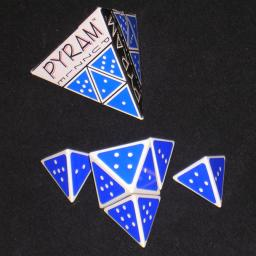The Pyram puzzle consists of four tetrahedra and one octahedron, and they can be stacked together to form one larger tetrahedron. The triangular sides of the pieces are have dots on them just like dice, with values from 1 to 6 as normal. The aim is to assemble them as a larger tetrahedron in such a way that the four values on each side sums up to 13. It is also possible to make each side sum to 12, 14, 15 or 16.

The diagram below shows the arrangement of the numbers on the five pieces: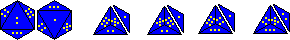This puzzle was made by the same company that made Pyrix, and Pyrus, namely Enpros Novelty Products, so the pieces and the packaging are of identical construction.

## Solutions:

The tetrahedral pieces all have two pairs of numbers that add to 7. Therefore you can rotate such a piece through 180 degrees to change each number x to 7-x. The interesting thing is that the octahedral piece also has number pairs arranged in such a way that it can be rotated to change its numbers x to 7-x.
The upshot of this is that if you have a solution where each side sums to N, you can turn each piece around to create a solution that sumes to 28-N. Therefore the solutions that sum to 12 are essentially the same as those that sum to 16, and similarly those that sum to 13 are the same as those that sum to 15.

There are 20 solutions that sum to 12.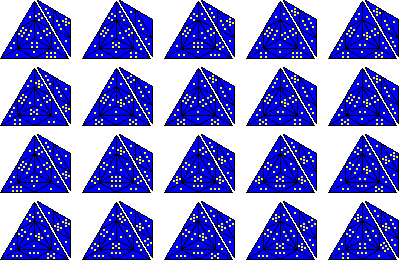There are 79 solutions that sum to 13.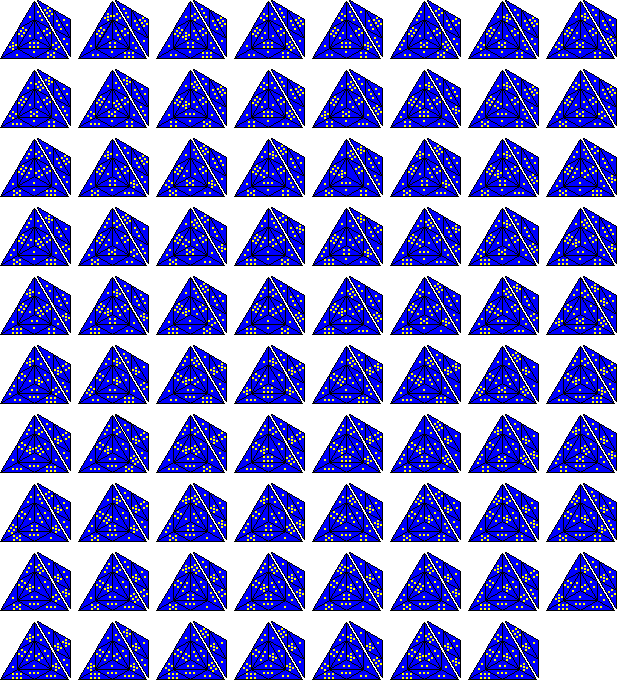There are 72 solutions that sum to 14. Half are shown below, the other half are the same with every number x replaced by 7-x.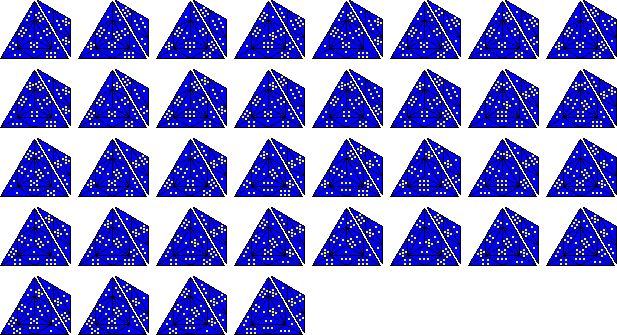There are 79 solutions that sum to 15, and 20 that sum to 16. These are not shown here, as they are the same as the solutions that sum to 13 and 12 respectively with every number x replaced by 7-x.